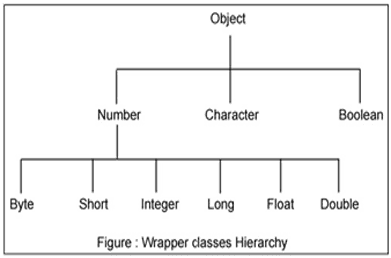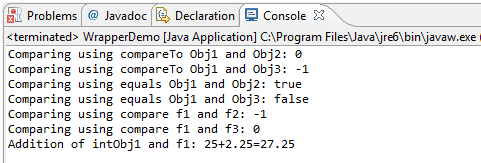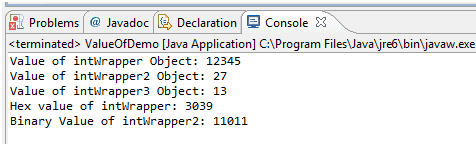# Java Wrapper Classes

## Description

Each of Java's eight primitive data types has a class dedicated to it. These are known as wrapper classes because they "wrap" the primitive data type into an object of that class. The wrapper classes are part of the java.lang package, which is imported by default into all Java programs.

The wrapper classes in java servers two primary purposes.

• To provide a mechanism to ‘wrap’ primitive values in an object so that primitives can do activities reserved for the objects like being added to ArrayList, Hashset, HashMap etc. collection.
• To provide an assortment of utility functions for primitives like converting primitive types to and from string objects, converting to various bases like binary, octal or hexadecimal, or comparing various objects.

The following two statements illustrate the difference between a primitive data type and an object of a wrapper class:

```int  x = 25;
Integer  y = new Integer(33);
```

The first statement declares an int variable named x and initializes it with the value 25. The second statement instantiates an Integer object. The object is initialized with the value 33 and a reference to the object is assigned to the object variable y.

Below table lists wrapper classes in Java API with constructor details.

Primitive Wrapper Class Constructor Argument
boolean Boolean boolean or String
byte Byte byte or String
char Character char
int Integer int or String
float Float float, double or String
double Double double or String
long Long long or String
short Short short or String

Below is wrapper class hierarchy as per Java APIAs explain in above table all wrapper classes (except Character) take String as argument constructor. Please note we might get NumberFormatException if we try to assign invalid argument in the constructor. For example to create Integer object we can have the following syntax.

```Integer intObj = new Integer (25);
Integer intObj2 = new Integer ("25");
```

Here in we can provide any number as string argument but not the words etc. Below statement will throw run time exception (NumberFormatException)

```Integer intObj3 = new Integer ("Two");
```

The following discussion focuses on the Integer wrapperclass, but applies in a general sense to all eight wrapper classes.

The most common methods of the Integer wrapper class are summarized in below table. Similar methods for the other wrapper classes are found in the Java API documentation.

Method Purpose
parseInt(s)

returns a signed decimal integer value equivalent to string s

toString(i) returns a new String object representing the integer i
byteValue() returns the value of this Integer as a byte
doubleValue() returns the value of this Integer as a double
floatValue() returns the value of this Integer as a float
intValue() returns the value of this Integer as an int
shortValue() returns the value of this Integer as a short
longValue() returns the value of this Integer as a long
int compareTo(int i) Compares the numerical value of the invoking object with that of i. Returns 0 if the values are equal. Returns a negative value if the invoking object has a lower value. Returns a positive value if the invoking object has a greater value.
static int compare(int num1, int num2) Compares the values of num1 and num2. Returns 0 if the values are equal. Returns a negative value if num1 is less than num2. Returns a positive value if num1 is greater than num2.
boolean equals(Object intObj) Returns true if the invoking Integer object is equivalent to intObj. Otherwise, it returns false.

Let’s see java program which explains few wrapper classes methods.

``````package WrapperIntro;
public class WrapperDemo {
public static void main (String args[]){
Integer intObj1 = new Integer (25);
Integer intObj2 = new Integer ("25");
Integer intObj3= new Integer (35);
//compareTo demo
System.out.println("Comparing using compareTo Obj1 and Obj2: " + intObj1.compareTo(intObj2));
System.out.println("Comparing using compareTo Obj1 and Obj3: " + intObj1.compareTo(intObj3));
//Equals demo
System.out.println("Comparing using equals Obj1 and Obj2: " + intObj1.equals(intObj2));
System.out.println("Comparing using equals Obj1 and Obj3: " + intObj1.equals(intObj3));
Float f1 = new Float("2.25f");
Float f2 = new Float("20.43f");
Float f3 = new Float(2.25f);
System.out.println("Comparing using compare f1 and f2: " +Float.compare(f1,f2));
System.out.println("Comparing using compare f1 and f3: " +Float.compare(f1,f3));
Float f = intObj1.floatValue() + f1;
System.out.println("Addition of intObj1 and f1: "+ intObj1 +"+" +f1+"=" +f );
}

}
```
```

Output:valueOf (), toHexString(), toOctalString() and toBinaryString() Methods:

This is another approach to creating wrapper objects. We can convert from binary or octal or hexadecimal before assigning a value to wrapper object using two argument constructor. Below program explains the method in details.

``````
package WrapperIntro;
public class ValueOfDemo {
public static void main(String[] args) {
Integer intWrapper = Integer.valueOf("12345");
//Converting from binary to decimal
Integer intWrapper2 = Integer.valueOf("11011", 2);
Integer intWrapper3 = Integer.valueOf("D", 16);
System.out.println("Value of intWrapper Object: "+ intWrapper);
System.out.println("Value of intWrapper2 Object: "+ intWrapper2);
System.out.println("Value of intWrapper3 Object: "+ intWrapper3);
System.out.println("Hex value of intWrapper: " + Integer.toHexString(intWrapper));
System.out.println("Binary Value of intWrapper2: "+ Integer.toBinaryString(intWrapper2));
}
}
```
```

Output:Summary

• Each of primitive data types has dedicated class in java library.
• Wrapper class provides many methods while using collections like sorting, searching etc.
﻿